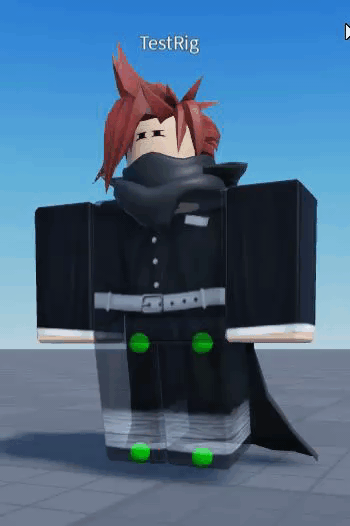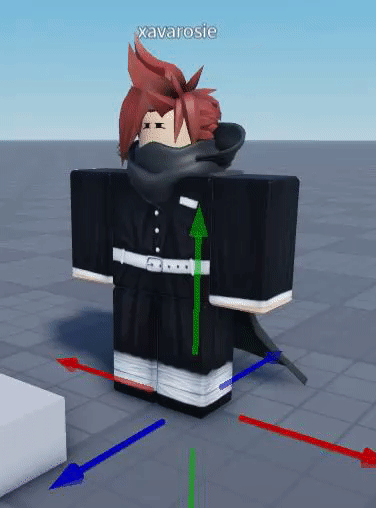# Can't make hard-coded inverse kinematics

Hello!
Recently, I’ve tried to make a hard-coded Inverse Kinematics script that only bends in one direction, like kneeling on the ground.

Keep in mind that the rig is R6, so its a bit harder to do this ` :/`

So far, I’ve tried to take the distance between two marked attachments parented to the torso and tried clamping the distance between 0 and 1, and then subtracting 1 by the variable. THEN multiplying that by 90 so that the knees start bending. It worked, but I can’t actually get the leg to look at the 2nd attachment.

It looks like this:

``````local ShowIKparts = true

local Character = script.Parent

local Torso = Character.Torso

local LeftLeg = Torso['Left Hip']

local LeftStart = LeftLeg.C0

local Left_FROM_att = Torso.IKleftTop

local Left_TO_att = Torso.IKleftBottom

local Left_MIDPOINT = (Left_FROM_att.Position+Left_TO_att.Position)/2

local RightLeg = Torso['Right Hip']

local RightStart = RightLeg.C0

local Right_FROM_att = Torso.IKrightTop

local Right_TO_att = Torso.IKrightBottom

local Right_MIDPOINT = (Right_FROM_att.Position+Right_TO_att.Position)/2

local leftDist = (Left_FROM_att.Position - Left_TO_att.Position).Magnitude

local rightDist = (Right_FROM_att.Position - Right_TO_att.Position).Magnitude

coroutine.resume(coroutine.create(function()

while wait() do

Right_TO_att.Position = Vector3.new(0.5, (math.sin(tick()*3))-2, 0)

Left_TO_att.Position = Vector3.new(-0.5, (math.sin(tick()*3))-2, 0)

end

end))

local IKlimits = {

AngleLimit = 70;

BendLimit = 0;

}

local RightOffset = (RightLeg.C0:Inverse()*CFrame.new(0.5,0,0)) * Right_FROM_att.CFrame

IKlimits.AngleLimit/=2

while wait() do

leftDist = (Left_TO_att.Position - Left_FROM_att.Position).Magnitude

rightDist = (Right_TO_att.Position - Right_FROM_att.Position).Magnitude

local PushLength_R = -(2-math.clamp(rightDist-IKlimits.BendLimit, 0, 2))*IKlimits.AngleLimit

local PushLength_L = (2-math.clamp(leftDist-IKlimits.BendLimit, 0, 2))*IKlimits.AngleLimit

RightLeg.C0 = RightIKcalc * CFrame.new(-0.5,3,0)

LeftLeg.C0 = LeftIKcalc * CFrame.new(0.5,3,0)

end
``````

The result comes out to this:This is what happens when I try to move it via X and Z:If you can help me find a way to get the Leg point towards a point AND start bending at a certain distance, I would be super thankful.

1 Like

Do you have the X values and the Z values in the CFrame accidentally switched?

Yes, my bad. I forgot to fix that. But that method is dead, and I need to make a new one that also makes the leg point at the IK target.

1 Like

Oh alright, fill me in on how it goes and if there are any new occuring issues!YES! GOOD NEWS! I figured it out!

The solution was to apply the orientation between IKFrom and IKTo to the motor, then add leg bending at a distance!

1 Like